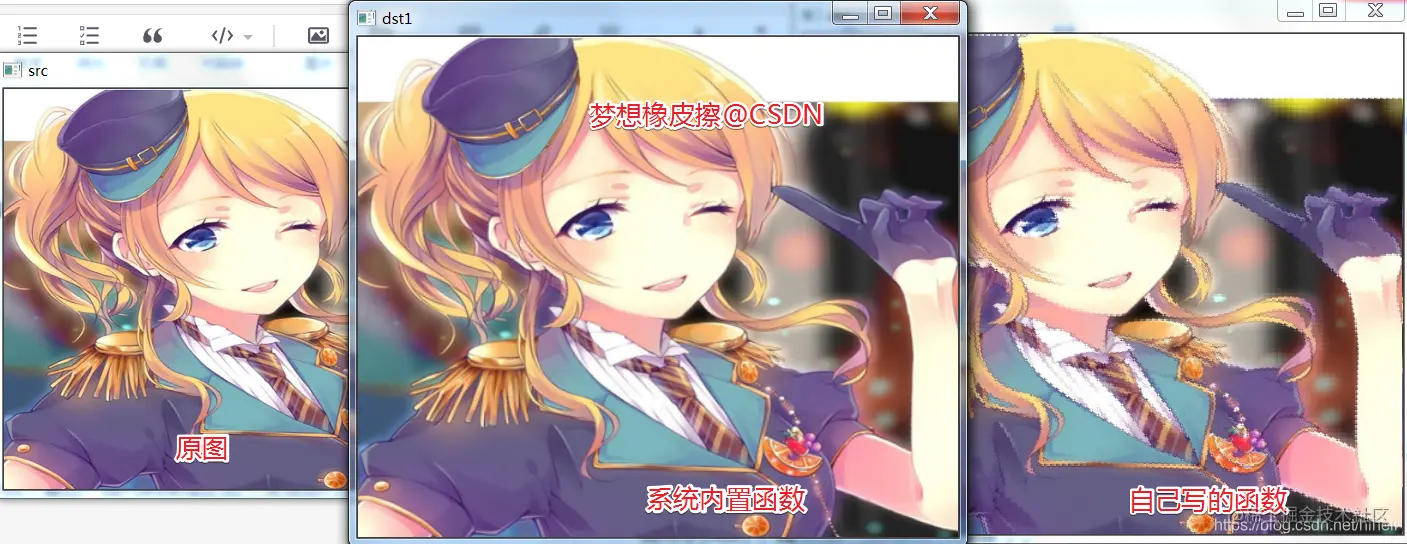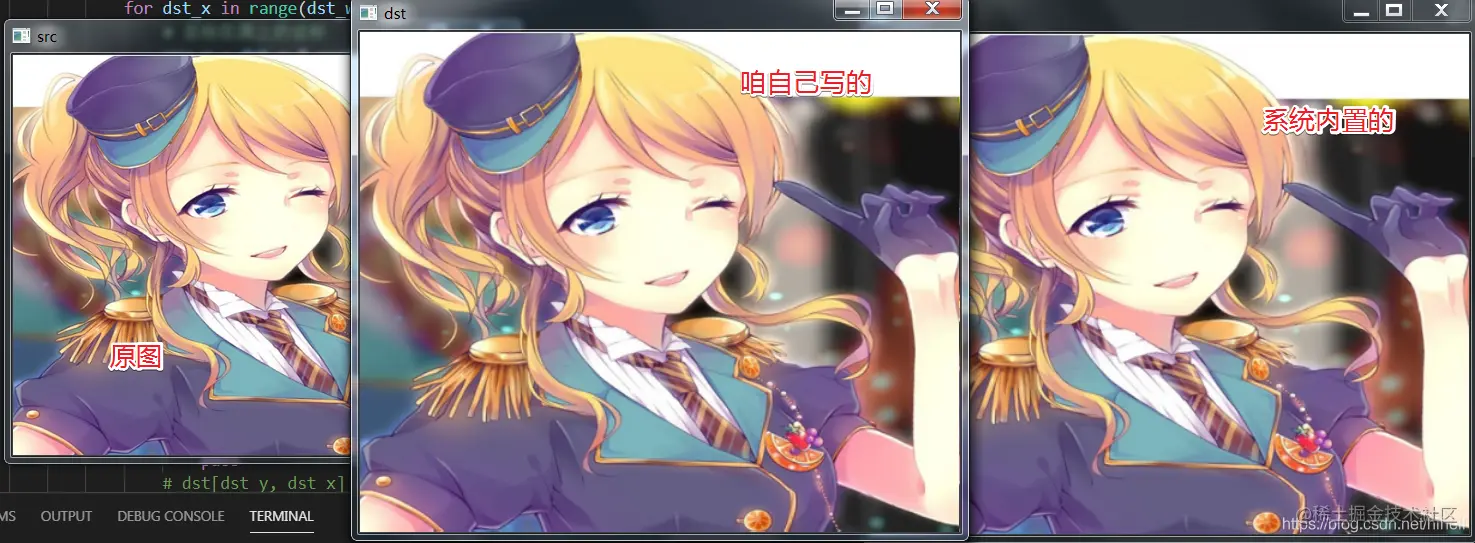# Optimization iteration of nearest neighbor interpolation and bilinear interpolation algorithm for Python OpenCV image

2022-01-30 05:10:13

Little knowledge , Great challenge ！ This article is participating in 「 A programmer must have a little knowledge 」 Creative activities

This article has participated in  「 Digging force Star Program 」 , Win a creative gift bag , Challenge creation incentive fund .

Python OpenCV 365 Day study plan , Go into the field of image with the eraser . This blog is the third in this series 43 piece .

## Basic knowledge

First make up for a small hole in yesterday's article , After the last piece of code is implemented , It is found that there are many jagged edges on the image after running .While wondering , There must be something wrong with the details of the code , When reviewing the code, I found a problem , Note the following codes ：

dst[dst_y, dst_x, n] = (1-u)*(1-v)*src[j, i, n]+u*(1-v) * src[j+1, i, n] + (1-u)*v*src[j, i+1, n] + u*v*src[j+1, i+1, n]
Copy code 

Comparison with formula , You can compare this detailed work by yourself . f(i+u,j+v) = (1-u)(1-v)f(i,j) + (1-u)vf(i,j+1) + u(1-v)f(i+1,j) + uvf(i+1,j+1)

The problem lies in the rows and columns of the image , Code u*(1-v) * src[j+1, i, n] + (1-u)*v*src[j, i+1, n] I wrote this part backwards ~ embarrassed

Change to the following code , Get it done , As like as two peas. .

dst[dst_y, dst_x, n] = (1-u)*(1-v)*src[j, i, n]+v*(1-u) * src[j+1, i, n] + (1-v)*u*src[j, i+1, n] + u*v*src[j+1, i+1, n]
Copy code## Algorithm optimization

Let's talk about algorithm optimization , We're still there This blog Dug a small hole , The final result of nearest neighbor interpolation algorithm is not satisfactory , When the image is magnified, there is a strong sawtooth .First migrate the previous code , Modify to the following format ：

import cv2 as cv
import numpy as np

def nearest_demo(src, multiple_y, multiple_x):
src_y, src_x, src_c = src.shape
tar_x, tar_y, tar_c = src_x*multiple_x, src_y*multiple_y, src_c
#  Generate a black target image
tar_img = np.zeros((tar_y, tar_x, tar_c), dtype=np.uint8)
print(tar_img.shape)
#  The value of the rendered pixel
#  Be careful  y  It's height ,x  It's the width
for y in range(tar_y-1):
for x in range(tar_x-1):
#  Calculate the new coordinates  (x,y)  Which value is the coordinate in the source diagram

src_y = round(y*src_y/tar_y)
src_x = round(x*src_x/tar_x)

tar_img[y, x] = src[src_y, src_x]

return tar_img

print(src.shape)
cv.imshow("src", src)
# dsize = (cols,rows)  chinese ,( Width , Height )
dst = cv.resize(src, (src.shape*2, src.shape
* 2), interpolation=cv.INTER_NEAREST)
cv.imshow("dst", dst)

new_dst = nearest_demo(src, 2, 2)
cv.imshow("new_dst", new_dst)

cv.waitKey(0)
cv.destroyAllWindows()
Copy code 

The first part of the optimization is about the center point , This part is serious , The eraser found a lot of information , They didn't explain everything very clearly , Basically, I skipped the key points , I'm paraphrasing it , If you have a good explanation , Please provide it to me in the comments area , The point is that 0.5 Pixel problem .

If the above code wants to implement and OpenCV Provide the same effect as the built-in function , Key modifications are as follows ：

srcy = round(y*src_y/tar_y)
srcx = round(x*src_x/tar_x)
#  Revised as follows
srcy = round((y+0.5)*src_y/tar_y-0.5)
srcx = round((x+0.5)*src_x/tar_x-0.5)
Copy code 

It was in this place that I was directly ignorant , Some blogs wrote srcX=dstX* (srcWidth/dstWidth)+0.5*(srcWidth/dstWidth-1) It's equivalent to adding... To the original floating-point coordinates 0.5*(srcWidth/dstWidth-1)

But there is no other explanation for this place , Some bloggers will compare two images and say that the geometric center of the source image and the target image should be aligned , But I just drew two pictures and some simple text descriptions , The excerpt is as follows ：

Suppose the source image is 3x3, The coordinates of the center point of this image are (1,1), The target image is 9x9, The coordinates of the center point are (4,4), The center point alignment should refer to (4,4) Align points to (1,1) spot , But according to srcx = round(x*src_x/tar_x) And srcy = round(y*src_y/tar_y) Formula calculation , The coordinates of the center point obtained are (1.333,1.333) Not at all (1,1), Lower right of the whole image , Now you need to align .

Let's make a simple calculation . The center point alignment assumes that the source image does not move in advance , Finally, there should be the following formula .

The source image src Center point coordinates $(\cfrac{M-1}{2},\cfrac{M-1}{2})$, Target image dst Center point coordinates $(\cfrac{N-1}{2},\cfrac{N-1}{2})$

Bring the above values into the formula ： $srcx=dstx*(\cfrac{srcwidth}{dstwidth})+K$

The formula is converted into $\cfrac{M-1}{2}=\cfrac{N-1}{2}*\cfrac{M}{N}+K$

Finally, we can get K The value of is $\cfrac{1}{2}*(\cfrac{M}{N}-1)$

$\cfrac{M}{N}=\cfrac{srcwidth}{dstwidth}$

therefore K In fact, it is equal to $K=\cfrac{1}{2}*(\cfrac{srcwidth}{dstwidth}-1)$

Corresponding to the code , It becomes the following ：

#  Before the change
src_y = round(dst_y*src_height/tar_height)
src_x = round(dst_x*src_width/tar_width)
#  After modification
src_y = round(dst_y*src_height/tar_height+1/2*(src_height/tar_height-1))
src_x = round(dst_x*src_width/tar_width+1/2*(src_height/tar_height-1))
Copy code 

Actually, you're here for a while , And you get the final result .

#  After modification
src_y = round((dst_y+0.5)*src_height/tar_height-0.5)
src_x = round((dst_x+0.5)*src_width/tar_width-0.5)
Copy code 

After the modification , The running feeling is better than the built-in effect of the system , Ha ha ha .The same optimization is copied into the bilinear interpolation algorithm , I can compare the effect of running .

About running speed , I also found some information , However, the current basic knowledge is not enough , Let's put it on hold for now , This series has 100 When , We're talking about .

## Eraser bars

I didn't think of , One 0.5 The small problem of pixels is so laborious .

I hope today's 1 You get something in an hour , I'll see you on our next blog ~